# Catch up on the latest AI articles# Released From ReLU, Use Smooth Activation Functions In Adversarial Learning!

3 main points
✔️ Adversarial learning generally improves the robustness of machine learning models but reduces accuracy.
✔️ The non-smooth nature of the activation function ReLU is found to inhibit adversarial learning.
✔️ Simply replacing ReLU with a smooth function improves robustness without changing computational complexity or accuracy.

written by Cihang XieMingxing TanBoqing GongAlan YuilleQuoc V. Le
(Submitted on 25 Jun 2020 (v1), last revised 11 Jul 2021 (this version, v2))

Subjects: Machine Learning (cs.LG); Computer Vision and Pattern Recognition (cs.CV); Neural and Evolutionary Computing (cs.NE)code：The images used in this article are from the paper, the introductory slides, or were created based on them.

## outline

An image that has been perturbed is called an adversarial example, and although it does not differ significantly from the original image to the human eye (note: the original image is often referred to as a clean image in the context of adversarial examples), it does change the inference results of the classification model, but it changes the inference result of the model.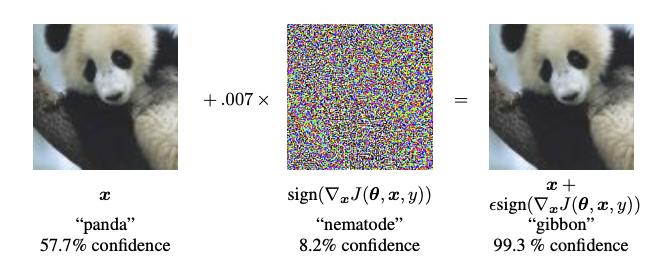The above figure is the hostile example, the perturbation is the image that looks like noise in the center, and the hostile example is the image on the right. As you can see, although the original image (left) and the hostile example are almost the same, the classification result has changed from panda to gibbon.

Hostile attacks against in-car AI that can recognize pedestrians or AI cameras that watch over infants can be used to deliberately cause unexpected behavior.

Adversarial learning is learning against adversarial attacks by including adversarial examples in the training dataset in advance. This allows the model to classify correctly even when adversarial examples are inputted, i.e., it improves the robustness. On the other hand, however, we found that the classification accuracy of the model itself decreases. Therefore, it is generally believed that model robustness and accuracy are incompatible.

In this paper, we have obtained a result that overturns the above common belief by analyzing the adversarial learning process. We found that the widely used activation function ReLU inhibits adversarial learning due to its non-smooth nature and that a smooth function can be used instead of ReLU to achieve both accuracy and robustness. smooth function instead of ReLU. We call this method smooth adversarial training (SAT).

## background

######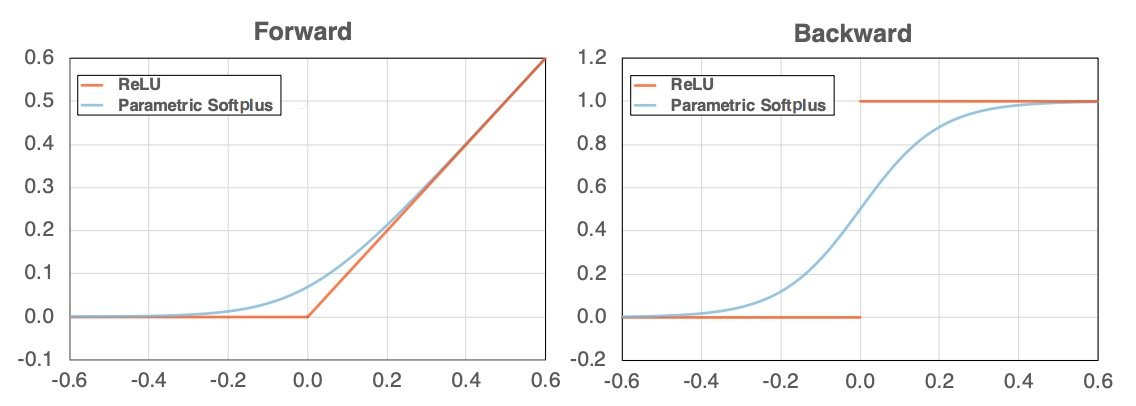As shown above, the graph of ReLU (orange on the left) changes its angle before and after x (abscissa) is zero. As a result, it becomes discontinuous if differentiated (orange in the right figure). In this paper, we show that adversarial learning does not work well due to this property and propose Smooth Adversarial Training (SAT), which substitutes ReLU for a smooth function.

## related research

Adversarial learning improves robustness by training the model on adversarial examples. In existing work, improving adversarial robustness requires either sacrificing accuracy on the source image (clean image) or increasing computational cost, such as gradient masking. This phenomenon is known as "No Free Lunch" (Note: there is no win-win solution) of adversarial robustness. It is called "No Free Lunch". However, in this paper, we show that smooth adversarial learning allows us to increase robustness for free.

Besides learning by adversarial examples, other methods to improve robustness have been reported, such as defensive distillation, gradient discretization, dynamic network architectures, randomized transformations, adversarial input denoising/purification, and so on. randomized transformations, adversarial input denoising/purification. However, it has been pointed out that these methods change the quality of the gradient and the gradient descent method may not work well.

### Weakening Adversarial Learning with ReLU

In this section, we show through a series of experiments whether ReLU inhibits adversarial learning in gradient computation (back propagation) paths, and conversely, whether smooth functions enhance adversarial learning. Adversarial learning can be thought of as an optimization problem, represented by the following equationwhere D is the data distribution, L is the loss function, θ is the network parameter, x is the training image, y is the correct label, ε is the perturbation, and S is the perturbation range. Note that S is set as small as possible so that the perturbation is not perceived by humans.

As shown in the above equation, adversarial learning is divided into two parts, one in the direction of maximizing the loss due to the adversarial example (inner) and the other in the direction of updating parameters to reduce the overall model loss (outer).

In this research, ResNet-50 is used as the base model and ReLU is used as the activation function by default. We use a single-step PGD attack (projected gradient descent attacker), which is a computationally inexpensive method to generate adversaries only once, instead of creating more and more powerful adversaries by learning. The adversary created by PGD-1 is trained.

PGD is an optimization technique used to compute perturbations. Perturbations generate adversarial examples, and the adversarial examples must fit within the training data distribution so that they look natural. Therefore, it is necessary to perform constrained optimization to "add optimal perturbations" within the range of "not unnatural", and PGD is used to do this (Note: PGD is often thought of as a method for generating adversarial examples because it is frequently used in the context of adversarial attacks, but it is an optimization technique).

Next, we use PGD-200 as the evaluation method (we also perform machine learning to create adversarial examples and update the perturbation 200 times so that the loss is maximized). Note that updating the attack 200 times is considered sufficient as a validation.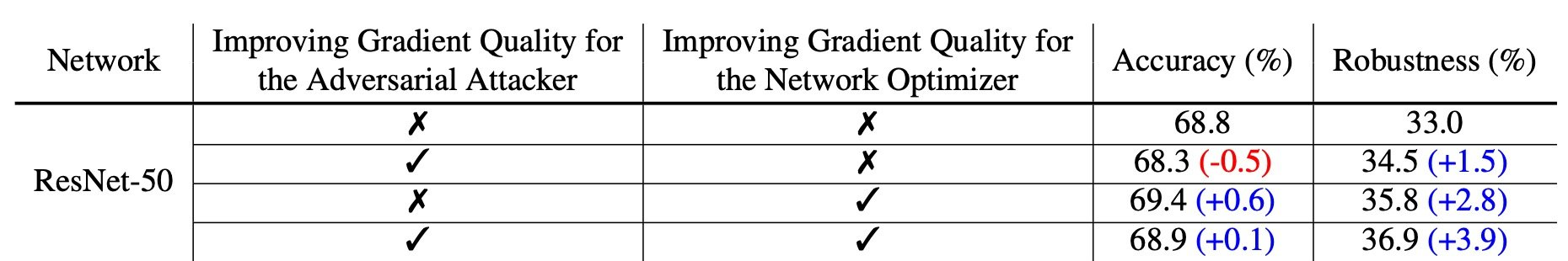The above is a summary of the results (Table 1). As mentioned earlier, adversarial learning is divided into (1) learning to strengthen the adversarial example (column 2, for the adversarial attacker) and (2) learning to reduce the overall model loss (column 3, for the network optimizer).

First, the PGD-200 attack on ResNet-50 without adversarial training yields an accuracy of 68.8% and robustness of 33%. In contrast, replacing ReLU with the Parametric Softplus function during network optimization improves robustness by 1.5%.The Parametric Softplus function is shown above. Alpha is an arbitrary number.The derivative is shown above; unlike ReLU, the derivative is also continuous (blue), as shown below.Adversarial learning is divided into two parts: 1) maximizing the loss due to adversarial examples (generating strong adversarial examples) and 2) minimizing the overall model loss (not allowing even strong adversarial examples to be misclassified). First, the authors point out that ReLU is unsuitable for (1) generating strong adversarial examples.

This is because generating adversarial examples requires gradient calculations, but the output of ReLU changes rapidly around x=0. As a result, ReLU weakens the process of learning stronger adversarial examples because the value changes significantly during perturbation calculations. Therefore, the authors proposed Parametric Softplus, a smooth function that approximates ReLU. Although α is arbitrary, it is empirically defined as α = 10 to better approximate ReLU.

Let's first look at the effect of gradient quality on (1) the adversary example computation (= inner maximization step) during training. The inner maximization step was expressed by the following equation.In this step, we generate a stronger adversary, but the generation of the adversary requires a gradient calculation. We use the Parametric Softplus function instead of ReLU.

To be precise, when learning to generate adversarial examples, we use ReLU for forwarding propagation and Parametric Softplus for back propagation. Then, when learning the model as a whole (adversarial learning), we use ReLU for both forward and back propagation. As a result, the robustness is improved by 1.5%, but the accuracy is reduced by 0.5% (Table 1).

In the previous section, we showed that using smooth functions during back propagation improves robustness.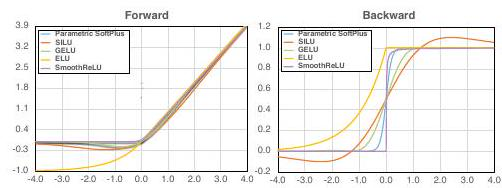Here we list several smooth functions and examine them in each.

### Softplus### silu### Gaussian Error Linear Unit (GELU)Φ is the cumulative distribution function of the standard normal distribution.

### Exponential Linear Unit (ELU)In this case, we assume α=1. This is because the derivatives are discontinuous when α is not 1.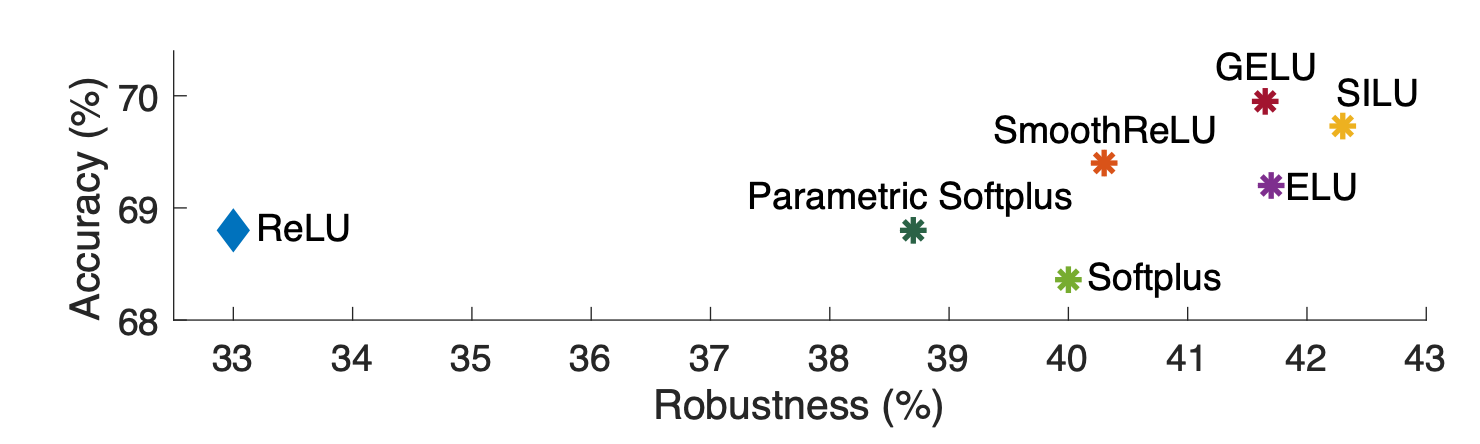The results are shown above (Figure 3). The most robust function is SILU, which achieves robustness of 42.3% and an accuracy of 69.7%. It is expected that a better smoothing function other than the one used in this study would improve the results even further.

## Conclusion

In this paper, we proposed Smooth Adversarial Training, in which the activation function is replaced by a smooth function in adversarial learning. Experimental results demonstrate the effectiveness of SAT and show that it significantly outperforms existing works in terms of accuracy and robustness.

If you have any suggestions for improvement of the content of the article,